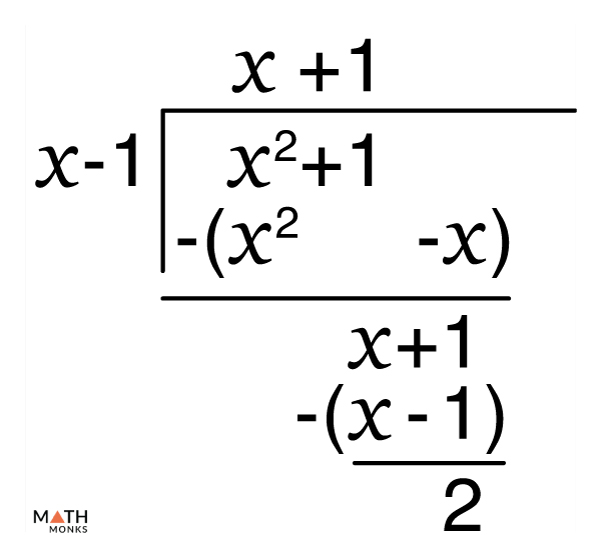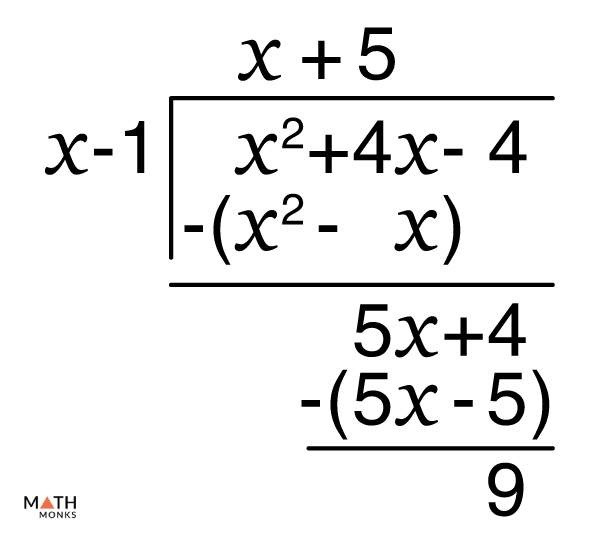# Oblique (Slant) Asymptote

An oblique or slant asymptote is a dashed line on a graph, describing the end behavior of a function approaching a diagonal line where the slope is neither zero nor undefined.

Thus, when either ${\lim _{x\rightarrow \infty }f\left( x\right)}$ or ${\lim _{x\rightarrow -\infty }f\left( x\right)}$ give the equation of a line mx + b, where m ≠ 0, then we say that the function f(x) has an oblique asymptote equal to mx + b, as shown below.

A function may have a horizontal or an oblique asymptote; it cannot have both. Like horizontal asymptotes, oblique asymptotes can cross the function. They can be negative and extend to infinity. Also, a function may have a maximum of two oblique asymptotes but can have infinitely many vertical asymptotes.

## How to Find Oblique Asymptotes

In general, for a function f(x), the oblique asymptote is a line l such that ${\lim _{x\rightarrow \pm \infty }\left( f\left( x\right) -l\left( x\right) \right) =0}$, or ${\lim _{x\rightarrow -\infty }\left( f\left( x\right) -l\left( x\right) \right) =0}$. To do this, we take l = mx + b and find m and b, which are the slope and the y-intercept, respectively, using these steps:

1. For m, divide f(x) by x and solve for the limit.
2. For b, subtract the value of mx from f(x) and solve for the limit.
3. Check for both positive and negative limits.

Thus, we get the equation of line l, which is the oblique asymptote of f(x). However, there are a few more direct methods to find the oblique asymptotes of various functions, which we will discuss below.

### For Rational Functions

Rational functions of the form ${f\left( x\right) =\dfrac{P\left( x\right) }{Q\left( x\right) }}$, where Q(x) ≠ 0, f(x) will have an oblique asymptote only when the degree of P(x) is greater than Q(x) by one.

We can get the oblique asymptote using either of two rules:

1. If the denominator can factorize the numerator, the oblique asymptote is the simplified form of the function.

For instance, the function ${f\left( x\right) =\dfrac{x^{2}-25}{x-5}}$ can be simplified to give f(x) = (x + 5). So, it has an oblique asymptote of y = x + 5.

1. For rational functions that cannot be simplified like above, we must first divide the numerator with the denominator and then compare the quotient with the line equation y = mx + b. We can use either synthetic division or long division for this.

Let us look at the function ${f\left( x\right) =\dfrac{2x^{2}+3x-1}{2x-1}}$. Since the denominator is not of the form (x + a), we cannot perform synthetic division. So, we must use long division to find the quotient.

We obtain (x + 2) as the quotient of the long division. The remainder is ignored. Thus, y = x +2 is the oblique asymptote of ${f\left( x\right) =\dfrac{2x^{2}+3x-1}{2x-1}}$.

Now let us take the function ${f\left( x\right) =\dfrac{2x^{2}+x-2}{x+2}}$. Here, the denominator corresponds to (x + a), so we may use synthetic division to find the quotient.

This method gives (2x + 3) as the quotient. Again, the remainder is ignored. Thus, we have y = 2x + 3 as the oblique asymptote of ${f\left( x\right) =\dfrac{2x^{2}+x-2}{x+2}}$.

### For Some Other Functions

• Hyperbolic functions of the form ${\dfrac{x^{2}}{a}-\dfrac{y^{2}}{b}=1}$ have two oblique asymptotes; ${y=\left( \dfrac{b}{a}\right) x}$ and ${y=\left( -\dfrac{b}{a}\right) x}$
• Polynomial functions of degree two or greater do not have oblique asymptotes.

## How to Graph Oblique Asymptotes

Once we get the equation of the oblique asymptote, the last step is graphing it. To do this, we follow these steps:

1. Find the y-intercept (0, b) by putting y = m × 0 + b.
2. Now find another point the graph passes through. Usually, this is the x-intercept.
3. Connect these two points and extend the line in both directions.

Here, the function ${f\left( x\right) =\dfrac{2x^{2}-4}{x+3}}$ has an oblique asymptote y = 2x – 6. The y and x-intercepts are (0, -6) and (3, 0). The graph thus looks like this:

## Solved Examples

Give the oblique asymptote of the function ${f\left( x\right) =\dfrac{x^{2}+1}{x-1}}$ .

Solution:We get the oblique asymptote using long division.
The quotient is (x + 1). Thus, the oblique asymptote is y = x +1.

Calculate the oblique asymptotes of the hyperbola ${\dfrac{x^{2}}{25}-\dfrac{y^{2}}{9}=1}$ .

Solution:

In the given hyperbola, a2 = 25 and b2 = 9. So, a = 5 and b = 3, respectively. The oblique asymptotes are thus y = (3/5)x and y = -(3/5)x.

Find the oblique asymptote of ${f\left( x\right) =\dfrac{x^{2}+4x+4}{x-1}}$ .

Solution:We will perform a long division to find the asymptote.
We get the quotient as (x + 5). The oblique asymptote is thus y = x + 5.